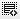## hey is there a formula to subtract on excel like we have =SUM() for addition0
and can we select a bunch of cells and minus it with another bunch of cells
is it also possible that you can do the same for multiplication and division0

Thats a very good question, but unfortunately not - there is no sum() equivalent function to subtract or multiply or divide.

One way to subtract a bunch of cells from another bunch of cells. Is to add both the group of cells separately and then subtract one sum from the other.

Click on thisicon to add code snippet.